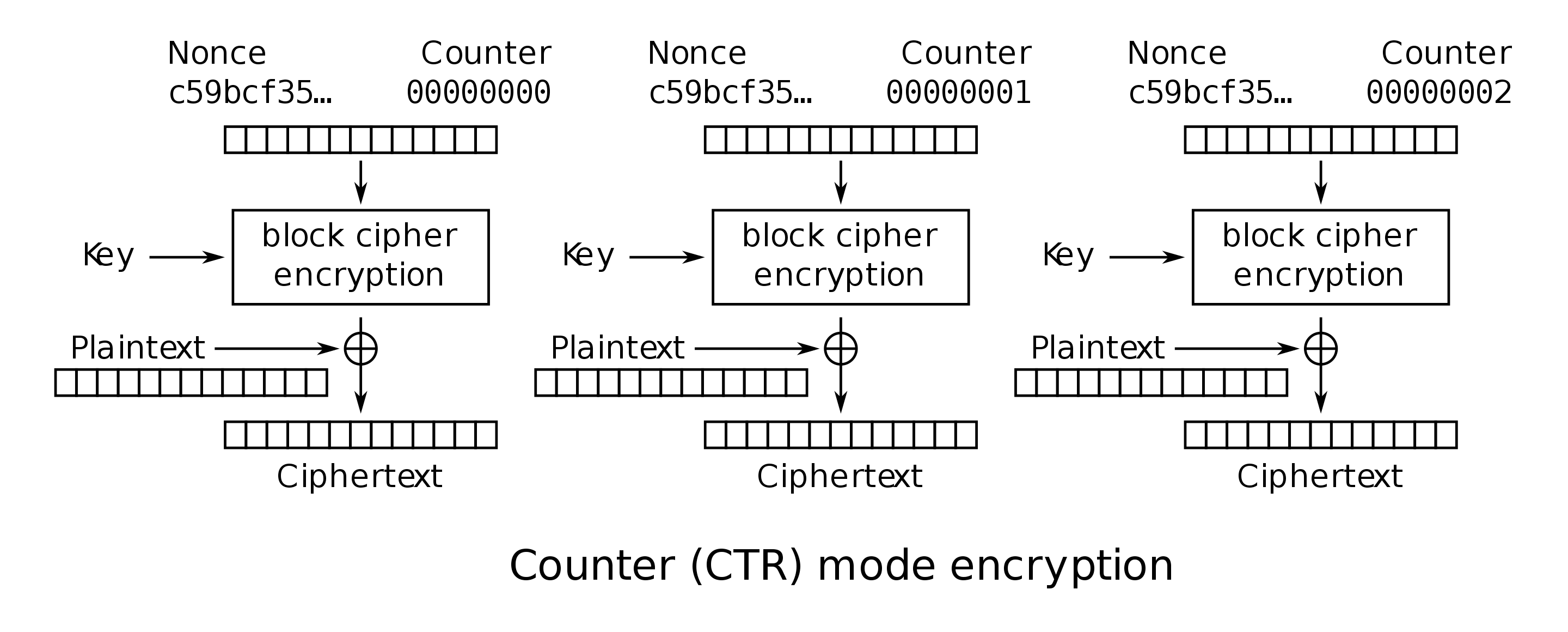# Discussion 03 Guide

## Question 2

a) Suppose you have access to function $R$ that takes a 128-bit seed $s$ and integers $n$, $m$ as input. $R$ outputs the $n$th (inclusive) through $m$th (exclusive) bits produced by the a pseudorandom generator $PRNG$ when it is seeded with seeds.

Use $R$ to make a secure symmetric-key encryption scheme. That is, define the key generation algorithm, the encryption algorithm, and the decryption algorithm.

Our inspiration for this algorithm comes from the one-time pad. A one-time pad is secure, as long as the key is never reused in an encryption. We can source a key for the encryption of each message from $RNG$ with $S$ being our key ($s = K$). Let $M$ be a 128-bit padded message. Our scheme is as follows:

1. Key Generation. Set $K \leftarrow_R \{0, 1\}^{128}$ (a random 128-bit number).
2. Encryption. We can prevent reuse of one-time pad keys by keeping track of the random numbers we have consumed from $RNG$ with a variable $j$.

Here, $||$ means concat, and we need to concat $j$ to our ciphertext for decryption. We also increment $j$ by $128$ after each encryption so our RNG gives us fresh random bits.

3. Decryption. Remember $x \oplus x = 0$. Let $\hat{C}$ be the ciphertext from $Enc$.

First, unpack $j$ from $\hat{C}$.

b) Explain how using a block cipher in counter (CTR) mode is similar to the scenario described above.

Below is the algorithm for CTR mode:There is a nonce (random number, also known as the initialization vector) that gets encrypted by a block cipher, producing a key for a one-time pad. The reason we have this nonce is that it makes the encryption non-deterministic as long as the nonce is used once per encryption. This is similar to the scheme above because we never reuse bits from $PRNG$.

One advantage of CTR mode is that, unlike our scheme, its computation can be easily parallelized because each partition’s encryption can be computed independently, given a nonce and a counter. Our scheme requires keeping track of $j$ across all encryptions, and also needs one bit from the PRNG for every bit in the message.

back up